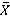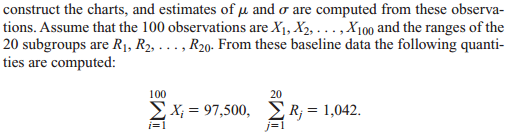Create an Account

Home / Questions / Suppose that the process mean shifts to 1011 in Problem 8 a What is the probability that t...

Suppose that the process mean shifts to 1011 in Problem 8 a What is the probability that the X­ chart will not indicate an out-of-control condition from a single subgroup sampling

Suppose that the process mean shifts to 1,011 in Problem 8.

a. What is the probability that the X­ chart will not indicate an out-of-control condition from a single subgroup sampling? (This is precisely the Type 2 error.)

b. What is the probability that the X­ chart will not indicate an out-of-control condition after sampling 20 subgroups?

Problem 8

Control charts for­ and R are maintained on the shear strength of spot welds. One hundred observations divided into subgroups of size five are used as a baseline toUsing this information, compute the values of three-sigma limits for both charts.

Jul 27 2020 View more View LessSubscribe To Get Solution